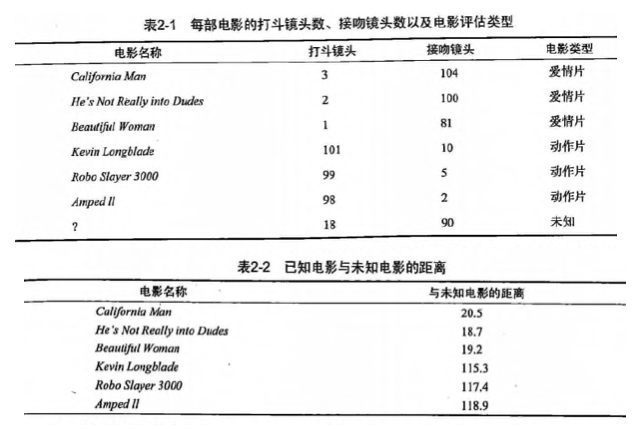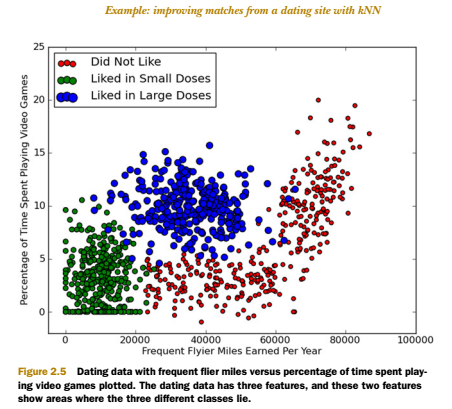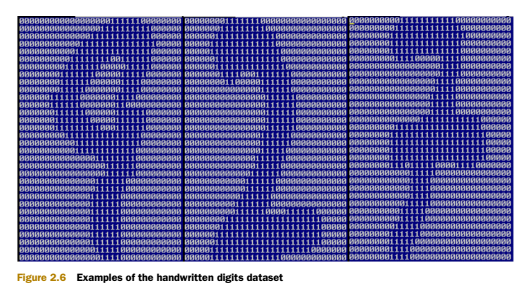# 第2章 k-近邻算法## KNN 概述

k-近邻（kNN, k-NearestNeighbor）算法是一种基本分类与回归方法，我们这里只讨论分类问题中的 k-近邻算法。

k 近邻算法的输入为实例的特征向量，对应于特征空间的点；输出为实例的类别，可以取多类。k 近邻算法假设给定一个训练数据集，其中的实例类别已定。分类时，对新的实例，根据其 k 个最近邻的训练实例的类别，通过多数表决等方式进行预测。因此，k近邻算法不具有显式的学习过程。

k 近邻算法实际上利用训练数据集对特征向量空间进行划分，并作为其分类的“模型”。 k值的选择、距离度量以及分类决策规则是k近邻算法的三个基本要素。

## KNN 场景

1. 动作片: 打斗次数更多
2. 爱情片: 亲吻次数更多现在根据上面我们得到的样本集中所有电影与未知电影的距离，按照距离递增排序，可以找到 k 个距离最近的电影。

knn 算法按照距离最近的三部电影的类型，决定未知电影的类型，而这三部电影全是爱情片，因此我们判定未知电影是爱情片。


## KNN 原理

KNN 工作原理

1. 假设有一个带有标签的样本数据集（训练样本集），其中包含每条数据与所属分类的对应关系。
2. 输入没有标签的新数据后，将新数据的每个特征与样本集中数据对应的特征进行比较。
1. 计算新数据与样本数据集中每条数据的距离。
2. 对求得的所有距离进行排序（从小到大，越小表示越相似）。
3. 取前 k （k 一般小于等于 20 ）个样本数据对应的分类标签。
3. 求 k 个数据中出现次数最多的分类标签作为新数据的分类。

KNN 通俗理解

KNN 开发流程

收集数据: 任何方法



KNN 算法特点

优点: 精度高、对异常值不敏感、无数据输入假定



## KNN 项目案例

### 项目案例1: 优化约会网站的配对效果

#### 项目概述

• 不喜欢的人
• 魅力一般的人
• 极具魅力的人

1. 工作日与魅力一般的人约会
2. 周末与极具魅力的人约会
3. 不喜欢的人则直接排除掉

#### 开发流程

收集数据: 提供文本文件

测试样本和非测试样本的区别在于:
测试样本是已经完成分类的数据，如果预测分类与实际类别不同，则标记为一个错误。



• 每年获得的飞行常客里程数
• 玩视频游戏所耗时间百分比
• 每周消费的冰淇淋公升数

40920    8.326976    0.953952    3
14488    7.153469    1.673904    2
26052    1.441871    0.805124    1
75136    13.147394    0.428964    1
38344    1.669788    0.134296    1


def file2matrix(filename):
"""
Desc:
导入训练数据
parameters:
filename: 数据文件路径
return:
数据矩阵 returnMat 和对应的类别 classLabelVector
"""
fr = open(filename)
# 获得文件中的数据行的行数
# 生成对应的空矩阵
# 例如: zeros(2，3)就是生成一个 2*3的矩阵，各个位置上全是 0
returnMat = zeros((numberOfLines, 3))  # prepare matrix to return
classLabelVector = []  # prepare labels return
fr = open(filename)
index = 0
# str.strip([chars]) --返回已移除字符串头尾指定字符所生成的新字符串
line = line.strip()
# 以 '\t' 切割字符串
listFromLine = line.split('\t')
# 每列的属性数据
returnMat[index, :] = listFromLine[0:3]
# 每列的类别数据，就是 label 标签数据
classLabelVector.append(int(listFromLine[-1]))
index += 1
# 返回数据矩阵returnMat和对应的类别classLabelVector
return returnMat, classLabelVector


import matplotlib
import matplotlib.pyplot as plt
fig = plt.figure()
ax.scatter(datingDataMat[:, 0], datingDataMat[:, 1], 15.0*array(datingLabels), 15.0*array(datingLabels))
plt.show()1 0.8 400 0.5 1
2 12 134 000 0.9 3
3 0 20 000 1.1 2
4 67 32 000 0.1 2

1) 线性函数转换，表达式如下:

y=(x-MinValue)/(MaxValue-MinValue)



2) 对数函数转换，表达式如下:

y=log10(x)

![对数函数图像](http://data.apachecn.org/img/AiLearning/ml/2.KNN/knn_1.png)


3) 反余切函数转换，表达式如下:

y=arctan(x)*2/PI

![反余切函数图像](http://data.apachecn.org/img/AiLearning/ml/2.KNN/arctan_arccot.gif)


4) 式(1)将输入值换算为[-1,1]区间的值，在输出层用式(2)换算回初始值，其中和分别表示训练样本集中负荷的最大值和最小值。

def autoNorm(dataSet):
"""
Desc:
归一化特征值，消除特征之间量级不同导致的影响
parameter:
dataSet: 数据集
return:
归一化后的数据集 normDataSet. ranges和minVals即最小值与范围，并没有用到

归一化公式:
Y = (X-Xmin)/(Xmax-Xmin)
其中的 min 和 max 分别是数据集中的最小特征值和最大特征值。该函数可以自动将数字特征值转化为0到1的区间。
"""
# 计算每种属性的最大值、最小值、范围
minVals = dataSet.min(0)
maxVals = dataSet.max(0)
# 极差
ranges = maxVals - minVals
normDataSet = zeros(shape(dataSet))
m = dataSet.shape
# 生成与最小值之差组成的矩阵
normDataSet = dataSet - tile(minVals, (m, 1))
# 将最小值之差除以范围组成矩阵
normDataSet = normDataSet / tile(ranges, (m, 1))  # element wise divide
return normDataSet, ranges, minVals


kNN 算法伪代码:

对于每一个在数据集中的数据点:
计算目标的数据点（需要分类的数据点）与该数据点的距离
将距离排序: 从小到大
选取前K个最短距离
选取这K个中最多的分类类别
返回该类别来作为目标数据点的预测值

def classify0(inX, dataSet, labels, k):
dataSetSize = dataSet.shape
#距离度量 度量公式为欧氏距离
diffMat = tile(inX, (dataSetSize,1)) – dataSet
sqDiffMat = diffMat**2
sqDistances = sqDiffMat.sum(axis=1)
distances = sqDistances**0.5

#将距离排序: 从小到大
sortedDistIndicies = distances.argsort()
#选取前K个最短距离， 选取这K个中最多的分类类别
classCount={}
for i in range(k):
voteIlabel = labels[sortedDistIndicies[i]]
classCount[voteIlabel] = classCount.get(voteIlabel,0) + 1
sortedClassCount = sorted(classCount.iteritems(), key=operator.itemgetter(1), reverse=True)
return sortedClassCount


kNN 分类器针对约会网站的测试代码

def datingClassTest():
"""
Desc:
对约会网站的测试方法
parameters:
none
return:
错误数
"""
# 设置测试数据的的一个比例（训练数据集比例=1-hoRatio）
hoRatio = 0.1  # 测试范围,一部分测试一部分作为样本
# 从文件中加载数据
datingDataMat, datingLabels = file2matrix('data/2.KNN/datingTestSet2.txt')  # load data setfrom file
# 归一化数据
normMat, ranges, minVals = autoNorm(datingDataMat)
# m 表示数据的行数，即矩阵的第一维
m = normMat.shape
# 设置测试的样本数量， numTestVecs:m表示训练样本的数量
numTestVecs = int(m * hoRatio)
print 'numTestVecs=', numTestVecs
errorCount = 0.0
for i in range(numTestVecs):
# 对数据测试
classifierResult = classify0(normMat[i, :], normMat[numTestVecs:m, :], datingLabels[numTestVecs:m], 3)
print "the classifier came back with: %d, the real answer is: %d" % (classifierResult, datingLabels[i])
if (classifierResult != datingLabels[i]): errorCount += 1.0
print "the total error rate is: %f" % (errorCount / float(numTestVecs))
print errorCount


def classifyPerson():
resultList = ['not at all', 'in small doses', 'in large doses']
percentTats = float(raw_input("percentage of time spent playing video games ?"))
ffMiles = float(raw_input("frequent filer miles earned per year?"))
iceCream = float(raw_input("liters of ice cream consumed per year?"))
datingDataMat, datingLabels = file2matrix('datingTestSet2.txt')
normMat, ranges, minVals = autoNorm(datingDataMat)
inArr = array([ffMiles, percentTats, iceCream])
classifierResult = classify0((inArr-minVals)/ranges,normMat,datingLabels, 3)
print "You will probably like this person: ", resultList[classifierResult - 1]


>>> classifyPerson()
percentage of time spent playing video games?10
frequent flier miles earned per year?10000
liters of ice cream consumed per year?0.5
You will probably like this person: in small doses


### 项目案例2: 手写数字识别系统

#### 开发流程

收集数据: 提供文本文件。

区别在于测试样本是已经完成分类的数据，如果预测分类与实际类别不同，
则标记为一个错误

数字，并完成数字识别，美国的邮件分拣系统就是一个实际运行的类似系统def img2vector(filename):
returnVect = zeros((1,1024))
fr = open(filename)
for i in range(32):
for j in range(32):
returnVect[0,32*i+j] = int(lineStr[j])
return returnVect


>>> testVector = kNN.img2vector('testDigits/0_13.txt')
>>> testVector[0,0:32]
array([0., 0., 0., 0., 0., 0., 0., 0., 0., 0., 0., 0., 0., 0., 1., 1., 1., 1., 0., 0., 0., 0., 0., 0., 0., 0., 0., 0., 0., 0., 0.])
>>> testVector[0,32:64]
array([0., 0., 0., 0., 0., 0., 0., 0., 0., 0., 0., 0., 1., 1., 1., 1., 1., 1., 1., 0., 0., 0., 0., 0., 0., 0., 0., 0., 0., 0., 0.])


def handwritingClassTest():
# 1. 导入训练数据
hwLabels = []
trainingFileList = listdir('data/2.KNN/trainingDigits')  # load the training set
m = len(trainingFileList)
trainingMat = zeros((m, 1024))
# hwLabels存储0～9对应的index位置， trainingMat存放的每个位置对应的图片向量
for i in range(m):
fileNameStr = trainingFileList[i]
fileStr = fileNameStr.split('.')  # take off .txt
classNumStr = int(fileStr.split('_'))
hwLabels.append(classNumStr)
# 将 32*32的矩阵->1*1024的矩阵
trainingMat[i, :] = img2vector('data/2.KNN/trainingDigits/%s' % fileNameStr)

# 2. 导入测试数据
testFileList = listdir('data/2.KNN/testDigits')  # iterate through the test set
errorCount = 0.0
mTest = len(testFileList)
for i in range(mTest):
fileNameStr = testFileList[i]
fileStr = fileNameStr.split('.')  # take off .txt
classNumStr = int(fileStr.split('_'))
vectorUnderTest = img2vector('data/2.KNN/testDigits/%s' % fileNameStr)
classifierResult = classify0(vectorUnderTest, trainingMat, hwLabels, 3)
print "the classifier came back with: %d, the real answer is: %d" % (classifierResult, classNumStr)
if (classifierResult != classNumStr): errorCount += 1.0
print "\nthe total number of errors is: %d" % errorCount
print "\nthe total error rate is: %f" % (errorCount / float(mTest))


## KNN 小结

KNN 是什么？定义: 监督学习？ 非监督学习？

KNN 是一个简单的无显示学习过程，非泛化学习的监督学习模型。在分类和回归中均有应用。

K, K的取值

### 算法: （sklearn 上有三种）

Brute Force 暴力计算/线性扫描

KD Tree 使用二叉树根据数据维度来平分参数空间。

Ball Tree 使用一系列的超球体来平分训练数据集。

N 数据集样本数量(number of samples)， D 数据维度 (number of features)

Brute Force: O[DN^2]

KD Tree: O[DN log(N)]

Ball Tree: O[DN log(N)] 跟 KD Tree 处于相同的数量级，虽然建树时间会比 KD Tree 久一点，但是在高结构的数据，甚至是高纬度的数据中，查询速度有很大的提升。

Brute Force: O[DN]

KD Tree: 当维度比较小的时候， 比如 D<20, O[Dlog(N)] 。相反，将会趋向于 O[DN]

Ball Tree: O[Dlog(N)]

Intrinsic Dimensionality(本征维数) 和 Sparsity（稀疏度）

Brute Force 的查询时间不受影响。

k的取值 (k 个邻点)

Brute Force 的查询时间基本不受影响。

k 在N的占比较大的时候，使用 Brute Force 比较好。

Number of Query Points （查询点数量， 即测试数据的数量）

metric/distance measure 可以选择。 另外距离 可以通过weight 来加权。

leaf size 对KD Tree 和 Ball Tree 的影响

Nearest Centroid Classifier